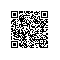# 第7章 集合类

val funlist: List<(Int) -> Boolean> =
listOf({ it -> it % 2 == 0 },
{ it -> it % 2 == 1 })


val list = listOf(1, 2, 3, 4, 5, 6, 7)
list.filter(funlist) // [2, 4, 6]
list.filter(funlist) //  [1, 3, 5, 7]


## 7.1 集合类概述

Kotlin的集合类分为：可变集合类（Mutable）与不可变集合类（Immutable）。

### 7.1.1 常用的3种集合类

• List 列表

List 列表的主要特征是其对象以线性方式存储，没有特定顺序，只有一个开头和一个结尾。列表在数据结构中可表现为：数组和向量、链表、堆栈、队列等。

• Set 集

Set 集是最简单的一种集合，它的对象不按特定方式排序，只是简单的把对象加入集合中，就像往口袋里放一堆溜溜弹珠。 Set 集中没有重复对象。

• Map 映射

Map 映射与Set 集或List 列表的区别是：Map 映射中每个项都是成对的。

Map 映射中存储的每个对象都有一个相关的关键字（Key）对象，关键字决定了 对象在映射中的存储位置，检索对象时必须提供相应的关键字，就像在字典中查单词一样。关键字是唯一的。

### 7.1.2 Kotlin 集合类继承层次

Kotlin 集合类继承层次

Iterable 父类。任何类继承这个接口就表示可以遍历序列的元素
MutableIterable 在迭代期间支持删除元素的迭代
Collection List和Set的父类接口。只读不可变
List 最常用的集合，继承Collection接口，元素有序，只读不可变
Set 元素无重复、无序。继承Collection接口。只读不可变
MutableSet 继承Set，支持添加和删除元素的Set
Map 存储 K-V（键-值）对的集合。在 Map 映射表中 key（键）是唯一的
MutableMap 支持添加和删除元素的Map

## 7.2 不可变集合类

List 列表分为只读不可变的 List 和 可变 MutableList （可写入删除数据）。List 集合类图如下

List 集合类图.png

Set 集也分为不可变 Set 和 可变 MutableSet（可写入删除数据） 。 Set 集合类图如下

Set 集合类图

Kotlin中的Map与List、Set一样，Map也分为只读Map和可变 MutableMap（可写入删除数据）。Map没有继承于Collection接口。其类图结构如下

Map 集合类图

## 7.3 创建集合类

Kotlin中使用 listOf() 、setOf()、mapOf() 创建不可变的 List列表、Set集、Map映射表；使用mutableListOf() 、mutableSetOf() 、mutableMapOf() 来创建可变的 MutableList 列表、MutableSet 集、MutableMap 映射表。代码示例如下

    val list = listOf(1, 2, 3, 4, 5, 6, 7)
val mutableList = mutableListOf("a", "b", "c")

val set = setOf(1, 2, 3, 4, 5, 6, 7)
val mutableSet = mutableSetOf("a", "b", "c")

val map = mapOf(1 to "a", 2 to "b", 3 to "c")
val mutableMap = mutableMapOf(1 to "X", 2 to "Y", 3 to "Z")


    val emptyList: List<Int> = listOf()
val emptySet: Set<Int> = setOf()
val emptyMap: Map<Int, String> = mapOf()


>>> val list = listOf()
error: type inference failed: Not enough information to infer parameter T in inline fun <T> listOf(): List<T>

val list = listOf()
^


## 7.4 遍历集合中的元素

List、Set 类继承了Iterable接口，里面扩展了forEach函数来迭代遍历元素；同样的 Map 接口中也扩展了forEach函数来迭代遍历元素。

    list.forEach {
println(it)
}

set.forEach {
println(it)
}

map.forEach {
println("K = ${it.key}, V =${it.value}") // Map里面的对象是Map.Entry<K, V>
}


public inline fun <T> Iterable<T>.forEach(action: (T) -> Unit): Unit
public inline fun <K, V> Map<out K, V>.forEach(action: (Map.Entry<K, V>) -> Unit): Unit


    list.forEachIndexed { index, value ->
println("list index = ${index} , value =${value}")
}

set.forEachIndexed { index, value ->
println("set index = ${index} , value =${value}")
}


public inline fun <T> Iterable<T>.forEachIndexed(action: (index: Int, T) -> Unit): Unit


Map的元素是Entry类型，由 entries属性持有

val entries: Set<Entry<K, V>>


 public interface Entry<out K, out V> {
public val key: K
public val value: V
}


>>> val map = mapOf("x" to 1, "y" to 2, "z" to 3)
>>> map
{x=1, y=2, z=3}
>>> map.entries
[x=1, y=2, z=3]


>>> map.entries.forEach({println("key="+ it.key + " value=" + it.value)})
key=x value=1
key=y value=2
key=z value=3


## 7.5 映射函数

map 函数

map 函数的代码示例如下

    val list = listOf(1, 2, 3, 4, 5, 6, 7)
val set = setOf(1, 2, 3, 4, 5, 6, 7)
val map = mapOf(1 to "a", 2 to "b", 3 to "c")

list.map { it * it } // [1, 4, 9, 16, 25, 36, 49]
set.map{ it + 1 } // [2, 3, 4, 5, 6, 7, 8]
map.map{ it.value + "$" } // [a$, b$, c$]


map 函数的签名如下

public inline fun <T, R> Iterable<T>.map(transform: (T) -> R): List<R>
public inline fun <K, V, R> Map<out K, V>.map(transform: (Map.Entry<K, V>) -> R): List<R>


val strlist = listOf("a", "b", "c")
strlist.map { it -> listOf(it + 1, it + 2, it + 3, it + 4) }


[[a1, a2, a3, a4], [b1, b2, b3, b4], [c1, c2, c3, c4]]


Kotlin中还提供了一个 flatten() 函数，效果是把嵌套的List结构“压平”，变成一层的结构，代码示例如下

strlist.map { it -> listOf(it + 1, it + 2, it + 3, it + 4) }.flatten()


[a1, a2, a3, a4, b1, b2, b3, b4, c1, c2, c3, c4]


flatMap 函数是把上面的先映射，再“压平”的两阶映射组合的结果，代码示例如下

strlist.flatMap { it -> listOf(it + 1, it + 2, it + 3, it + 4) }


[a1, a2, a3, a4, b1, b2, b3, b4, c1, c2, c3, c4]


## 7.6 过滤函数

data class Student(var id: Long, var name: String, var age: Int, var score: Int){
override fun toString(): String {
return "Student(id=$id, name='$name', age=$age, score=$score)"
}
}


    val studentList = listOf(
Student(1, "Jack", 18, 90),
Student(2, "Rose", 17, 80),
Student(3, "Alice", 16, 70)
)


studentList.filter { it.age >= 18 }


[Student(id=1, name='Jack', age=18, score=90)]


studentList.filter { it.score < 80 }


[Student(id=3, name='Alice', age=16, score=70)]


val list = listOf(1, 2, 3, 4, 5, 6, 7)
list.filterIndexed { index, it -> index % 2 == 0 && it > 3 }  // [5, 7]


filterIndexed 函数签名如下

public inline fun <T> Iterable<T>.filterIndexed(predicate: (index: Int, T) -> Boolean): List<T>


## 7.7 排序函数

val list = listOf(1, 2, 3, 4, 5, 6, 7)
val set = setOf(1,3,2)

list.reversed() // [7, 6, 5, 4, 3, 2, 1]
set.reversed() // [2, 3, 1]


public fun <T> Iterable<T>.reversed(): List<T> {
val list = toMutableList()
list.reverse()
return list
}

public fun <T> MutableList<T>.reverse(): Unit {
java.util.Collections.reverse(this)
}


>>> list.sorted()
[1, 2, 3, 4, 5, 6, 7]
>>> set.sorted()
[1, 2, 3]


Kotlin的这个 sorted() 函数也是直接调用的 Java 的API 来实现的，相关代码如下

public fun <T : Comparable<T>> Iterable<T>.sorted(): List<T> {
if (this is Collection) {
if (size <= 1) return this.toList()
@Suppress("UNCHECKED_CAST")
return (toTypedArray<Comparable<T>>() as Array<T>).apply { sort() }.asList()
}
}


public fun <T> Array<out T>.sort(): Unit {
if (size > 1) java.util.Arrays.sort(this)
}


## 7.7 元素去重

val dupList = listOf(1, 1, 2, 2, 3, 3, 3)
dupList.distinct() // [1, 2, 3]


Kotlin中集合类中还提供了许多功能丰富的API，此处不一一介绍。更多可以参考官方API文档：http://kotlinlang.org/api/latest/jvm/stdlib/kotlin.collections/index.html

## 本章小结使用钉钉扫一扫加入圈子
+ 订阅